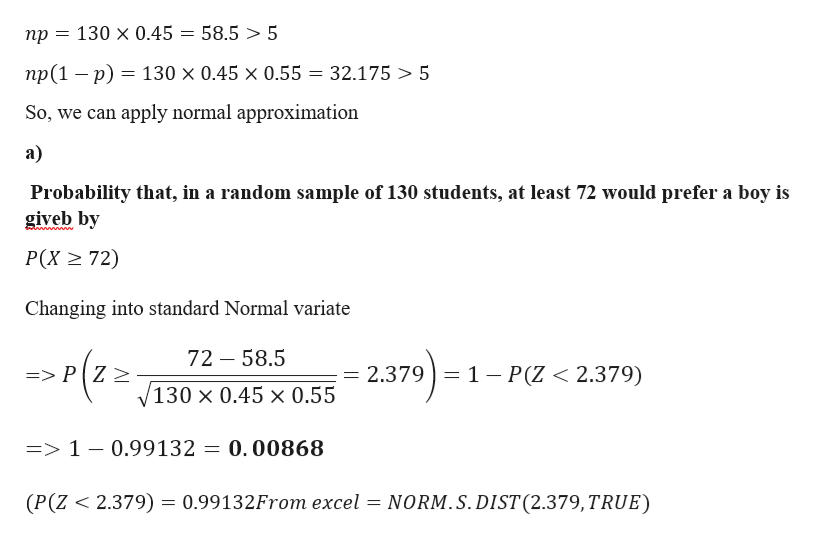# In a recent​ poll, 45​% of survey respondents said​ that, if they only had one​ child, they would prefer the child to be a boy. Suppose you conducted a survey of 130 randomly selected students on your campus and find that 72of them would prefer a boy. ​(a) Use the normal approximation to the binomial to approximate the probability​ that, in a random sample of 130 ​students, at least 72would prefer a​ boy, assuming the true percentage is 45​%.​(b) Does this result contradict the​ poll? Explain.

Question
75 views
In a recent​ poll, 45​% of survey respondents said​ that, if they only had one​ child, they would prefer the child to be a boy. Suppose you conducted a survey of 130 randomly selected students on your campus and find that 72
of them would prefer a boy.

​(a) Use the normal approximation to the binomial to approximate the probability​ that, in a random sample of 130 ​students, at least 72
would prefer a​ boy, assuming the true percentage is 45​%.
​(b) Does this result contradict the​ poll? Explain.
check_circle

Step 1

Given data

p= 0.45

n =130

Checking the validity of normal approximationhelp_outlineImage Transcriptionclose130 x 0.45 = 58.5 > 5 np np(1 p) 130 x 0.45 x 0.55 32.175 5 So, we can apply normal approximation а) Probability that, in a random sample of 130 students, at least 72 would prefer a boy is giveb by wwwwwe P(X 72) Changing into standard Normal variate 72 58.5 )-1 2.379 1 P(Z < 2.379) => P Z 2 130 x 0.45 x 0.55 => 1 - 0.99132 = 0.00868 (P(Z 2.379) = 0.99132From excel = NORM.S.DIST (2.379,TRUE) fullscreen
Step 2

b)

considering the significance level 0.05

probability = 0.00868 < 0.05...

### Want to see the full answer?

See Solution

#### Want to see this answer and more?

Solutions are written by subject experts who are available 24/7. Questions are typically answered within 1 hour.*

See Solution
*Response times may vary by subject and question.
Tagged in

### Other